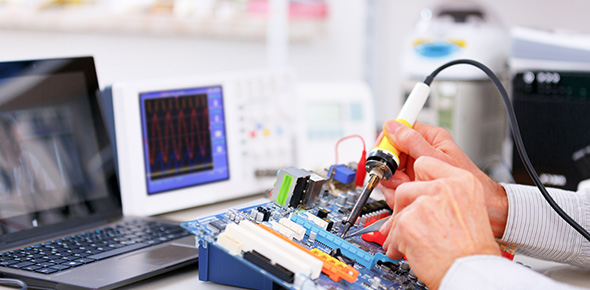# RAM And ROM Trivia Quiz

16 Questions | Total Attempts: 245SettingsIf you like answering specifically designed questions that touch on RAM and ROM trivia then try out this quiz. All computer geeks, this is for you. It is quite simple therefore it will be easy to answer.

Related Topics
• 1.
Explain the difference between ROM and RAM.  (2 marks)
• 2.
State whether each statement is True or False A touch screen is necessary on home computers
• A.

True

• B.

False

• 3.
State whether each statement is True or False The data on a DVD may be changed
• A.

True

• B.

False

• 4.
State whether each statement is True or False Supermarkets use bar-code readers
• A.

True

• B.

False

• 5.
State whether each statement is True or False MICR is used in banking
• A.

True

• B.

False

• 6.
State whether each statement is True or False ROM is a volatile storage medium
• A.

True

• B.

False

• 7.
Both RAM and ROM are used in computers. (a)     State what ROM is. (b)     Give one example of when ROM is used.
• 8.
Select whether the  statement is true or false. A GUI uses icons
• A.

True

• B.

False

• 9.
Select whether the  statement is true or false. DVD players use microprocessors
• A.

True

• B.

False

• 10.
Select whether the  statement is true or false. MICR is used to read bar codes
• A.

True

• B.

False

• 11.
Select whether the  statement is true or false. OCR is used to recognise scanned text
• A.

True

• B.

False

• 12.
Select whether the  statement is true or false. ROM loses its contents when the computer is switched off
• A.

True

• B.

False

• 13.
A computer has two types of memory, RAM and ROM. What does RAM stand for?
• 14.
A computer has two types of memory, RAM and ROM. What is RAM used for?
• 15.
A computer has two types of memory, RAM and ROM. What does ROM stand for?